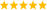# LabVIEW

cancel
Showing results for
Did you mean:

Solved!
Go to solution
Highlighted

## interpolate 1d array

Is this is interpolation array works as per Newton-Gregory forward interpolation formula.As i am not getting exact value in programming.kindly look into the attached pic for reference...as per formula i am getting 214.6875...but using programming i am getting 231.5...can anyone help me

Message 1 of 8
(1,672 Views)

## Re: interpolate 1d array

Hi sanjay,

did you read the help for this funtion?

With x=0.5 you get the mean of the array element 0 and 1: 225 + 0.5 * (238-225) = 231.5…

Best regards,
GerdW
CLAD expired, using LV2011SP1 + LV2017 (+LV2019 sometimes) on Win7+cRIO
Kudos are welcomeMessage 2 of 8
(1,652 Views)

## Re: interpolate 1d array

Hi Gerd,

as per  that function in LV what they have defined ,the answer is correct but ,in terms of mathematical equation ,i found answer was incorrect.

as per Newton-Gregory forward interpolation formula.......Interpolation function is derived from mathematics ,so when i started working theortically ,answer was not matching.kidly look into the attached pdf for reference

Thanks&Regards

Sanjay k

Message 3 of 8
(1,642 Views)

## Re: interpolate 1d array

Hi sanjay,

as per  that function in LV what they have defined ,the answer is correct but ,in terms of mathematical equation ,i found answer was incorrect.

In which way should the result be incorrect? Interpolate1DArray uses a simple linear interpolation as is clearly stated in the help!

as per Newton-Gregory forward interpolation formula

What has "Newton-Gregory" to do with Interpolate1DArray?

Best regards,
GerdW
CLAD expired, using LV2011SP1 + LV2017 (+LV2019 sometimes) on Win7+cRIO
Kudos are welcomeMessage 4 of 8
(1,639 Views)

## Re: interpolate 1d array

HI Gerd,

can u explain me ....about interpolate and threshold array ....in terms of mathematical equation...bit confused how it works

Regards

sanjay k

Message 5 of 8
(1,616 Views)
Solution
Accepted by topic author sanjayks066

## Re: interpolate 1d array

It is linear interpolation, very basic algebra.

For a given X between x0 and X1, you'll get the value of Y = Y0+ (Y1 -Y0)*(X-X0)/(X1-X0)

Message 6 of 8
(1,613 Views)

thanks Ravens

Message 7 of 8
(1,607 Views)

## Re: interpolate 1d array

The word "interpolation" is quite generic and does not specify any particular algorithm. There is "interpolate 1D array" that does a linear interpolation, but LabVIEW also has quite a few more advanced 1D and 2D interpolation algorithms in the interpolation palette. Maybe what you are looking for is in there, and if not, you can write your own and wrap it into a subVI. Looks like you have all the required formulas. Good luck! 😄LabVIEW Champion. It all comes together inGCentralMessage 8 of 8
(1,581 Views)